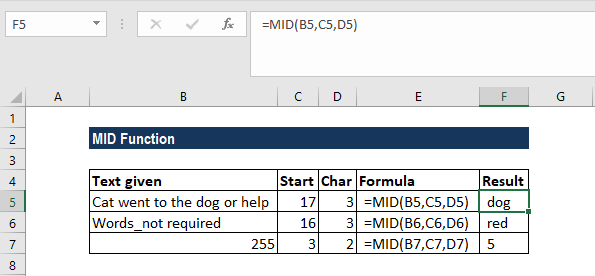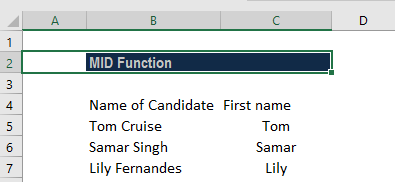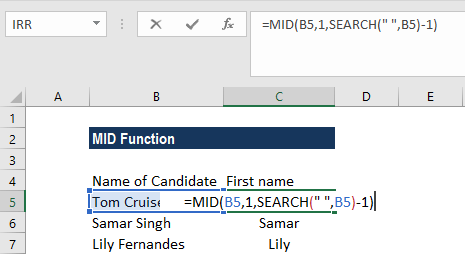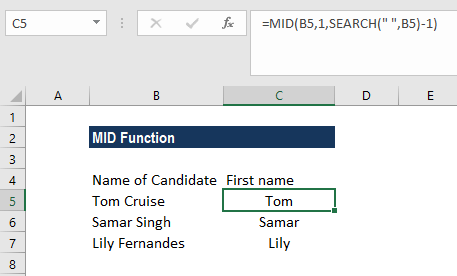# MID Function

Returns a specified number of characters from the middle of a given text string

## What is the MID Function?

The MID function is categorized under Excel TEXT functions. The function will return a specified number of characters from the middle of a given text string.

While doing financial analysis, the MID function can be useful if we wish to extract characters from the middle of a specific text. Generally, it is used by combining it with other functions such as VALUE, SUM, COUNT, DATE, DAY, etc.

### Formula

=MID(text,start_num,num_chars)

The MID function uses the following arguments:

1. Text (required argument) – The original text string.
2. Start_num (required argument) – This is an integer that specifies the position of the first character that you want to be returned.
3. Num_chars (required argument) – Specifies the number of characters, starting with start_num, to be returned from the start of the given text. It is the number of characters to be extracted.

### How to use the MID Function in Excel?

As a worksheet function, MID can be entered as part of a formula in a cell of a worksheet. To understand the uses of the function, let us consider an example:

#### Example 1

Suppose we wish to find certain characters from the data below:

• Cat went to the dog or help
• Words_not required
• 255

The results in Excel are shown in the screenshot below:In the first cell, we extracted characters 17-20. When we gave a number, MID extracted the character according to the arguments given above.

#### Example 2

Assume we extracted the first and last name of candidates separated by space. If we want, we can extract the first name using MID with the SEARCH function. We are given the data below:The formula to use is:We will get the results below:In the above formula, the SEARCH function is used to scan the original string for the space character (” “) and return its position, from which we subtract 1 to avoid trailing spaces. We then use the MID function to return a substring beginning with the first character and up to the character preceding the space, thus fetching the first name.

We can also use the MID formula to get the last name. To extract the last name from A2, we can use the formula below:

=TRIM(MID(A2,SEARCH(” “,A2),LEN(A2)))

### A few things to remember:

1. #VALUE! error – Occurs if the given [num_chars] argument is less than 0 or the given start_num argument is less than 1.
2. Dates are stored in Excel as numbers, and it is only the cell formatting that makes them appear as dates in our spreadsheet. Hence, if we attempt to use the MID function on a date, it will return the middle characters of the number that represents that date.

For example, 01/01/1980 is represented by the number 29221, so applying the function to a cell containing the date 01/01/1980 (and requesting that 3 characters be returned, starting from the second character) will result in a returned value of “922.” If we wish to use this on dates, we can combine it with the DAY, DATE, MONTH or YEAR functions. Otherwise, we can convert the cells containing dates to text using Excel’s Text to Columns tool.

Thanks for reading CFI’s guide to important Excel functions! By taking the time to learn and master these functions, you’ll significantly speed up your financial analysis. To learn more, check out these additional CFI resources:

• Excel Functions for Finance